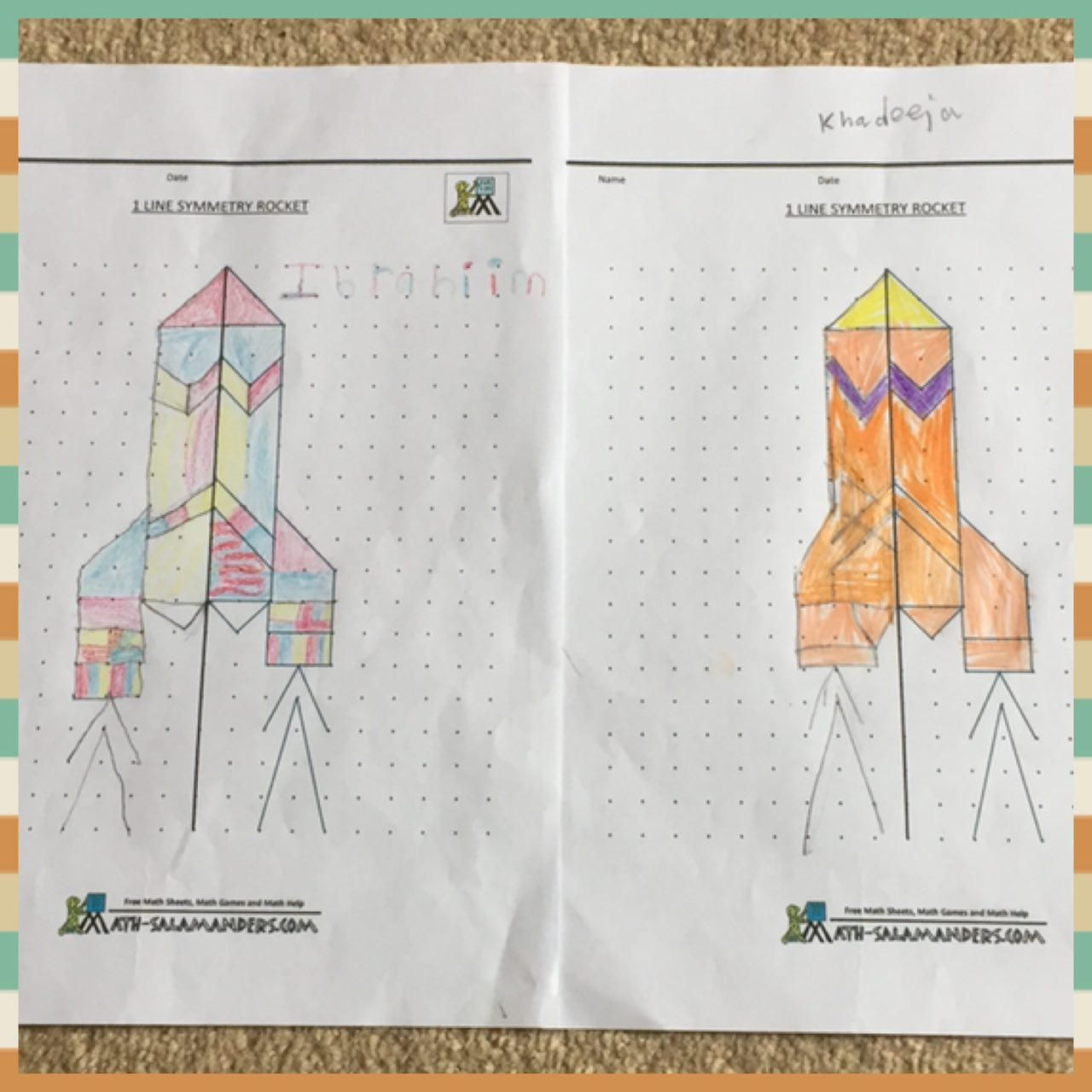# ?Lines of Symmetry?

Year 2 students have been learning about Symmetry within their Maths lessons.

When something is symmetrical it means it is the same on both sides.

The students were given a shape and asked to draw lines of symmetry on them. To help understand the concept and how to find the line of symmetry, the children were presented with shapes to fold in half so that they could see clearly that one side was the same as the other.

A shape has symmetry if a central dividing line (a mirror line) can be drawn on it that shows that both sides of the shape are exactly the same.